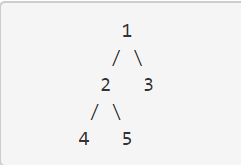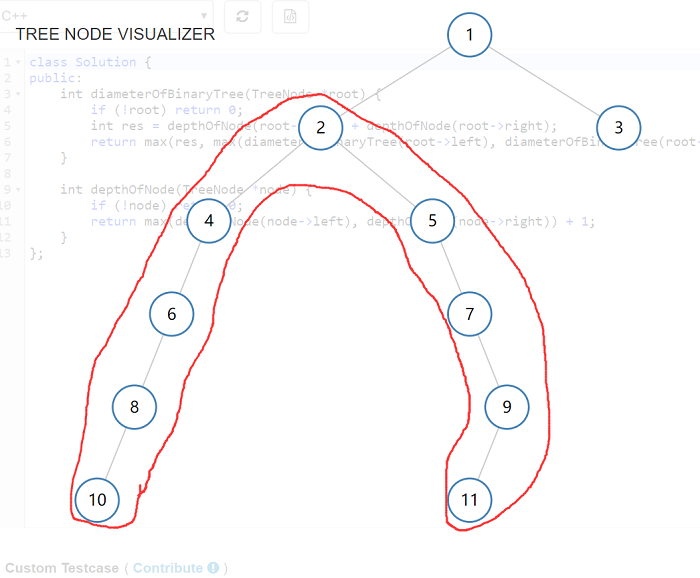# 一、引言Given a binary tree, you need to compute the length of the diameter of the tree. The diameter of a binary tree is the length of the longest path between any two nodes in a tree. This path may or may not pass through the root.

Exmaple:
Given a binary treeReturn 3, which is the length of the path [4, 2, 1, 3] or [5, 2, 1, 3].

Note:
The length of path between two nodes is represented by the number of edges between them.# 二、我之所思：找圆心

diameter

1. 如何遍历所有节点：使用递归方法遍历所有节点，去计算其左子树深度和右子树深度之和，找到和最大的一个返回和计算即可

2. 如何计算一个节点的深度：计算一个节点的深度，我们可以同样使用递归遍历，每层递归计算每一个节点左子树和右子树的深度之中，取其中最大的一个，并且要加 1（因为一层递归相当于就是递进一层深度）

// my solution 6 , runtime = 26 ms
class Solution6 {
public:
int diameterOfBinaryTree(TreeNode *root) {
traverseBinaryTree(root);
int diameter = 0;
for (auto i : list) {
int sumary = 0;
sumary = depthOfNode(i->left) + depthOfNode(i->right);
diameter = sumary > diameter ? sumary : diameter;
}
return diameter;
}

void traverseBinaryTree(TreeNode *node) {
if (!node) return;
list.push_back(node);
traverseBinaryTree(node->left);
traverseBinaryTree(node->right);
}

int depthOfNode(TreeNode *node) {
if (!node) return 0;
return max(depthOfNode(node->left), depthOfNode(node->right)) + 1;
}

private:
vector<TreeNode*> list;
};

1. 三个函数：这一块有三个函数，主要函数是 diameterOfBinaryTree() ，用来接受题目输入并且输出结果；在这个函数中调用 traverseBinaryTree() 函数来实现了二叉树的遍历，将二叉树中所有的节点都放置到一个存储容器 list 中，以便后面使用；最后遍历这个容器 list（也就是遍历所有的树节点），挨个计算每个节点的左子树深度和右子树深度之和，找到其中最大的一个返回

2. 二叉树节点存储容器：这个容器被定义为 std::vector<TreeNode*> 类型，用来方便后面遍历求节点深度

3. 递归求节点深度：求一个节点的深度，必然是求其左子树和右子树中深度较大的一个的深度 + 1；按照这个规律递归即可

# 三、分析的胜利：最高票答案的妙处

// perfect solution , runtime = 26 ms
class Solution7 {
public:
int diameterOfBinaryTree(TreeNode *root) {
if (!root) return 0;
int res = depthOfNode(root->left) + depthOfNode(root->right);
return max(res, max(diameterOfBinaryTree(root->left), diameterOfBinaryTree(root->right)));
}

int depthOfNode(TreeNode *node) {
if (!node) return 0;
return max(depthOfNode(node->left), depthOfNode(node->right)) + 1;
}
};

We can solve this problem with two different cases:
1. If the longest path will include the root node, then the longest path must be the depth(root->right) + depth(root->left)
2. If the longest path does not include the root node, this problem is divided into 2 sub-problem: set left chid and right child as the new root separately, and repeat step1.

1. 最长路径经过根节点：那么根节点的左子树的深度和右子树的深度就是我们的结果
2. 最长路径没有经过根节点：这个问题就分为两个子问题，分别设置新的根节点为其左子节点和右子节点，然后重复步骤 1

return max(res, max(diameterOfBinaryTree(root->left), diameterOfBinaryTree(root->right)));

# 四、总结

To be Stronger!